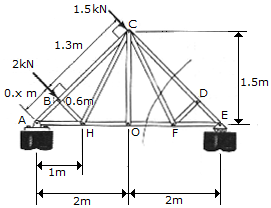# Engineering Mechanics - Structural Analysis - Discussion

### Discussion :: Structural Analysis - General Questions (Q.No.7)

7.Determine the force in members GF, CF, CD of the symmetric roof truss and indicate whether the members are in tension or compression.

 [A]. CD = 1.783 kN C, CF = 0, GF = 1.427 kN T [B]. CD = 3.48 kN C, CF = 00904 kN T, GF = 1.783 kN T [C]. CD = 2.79 kN C, CF = 0.723 kN T, GF = 1.427 kN T [D]. CD = 2.23 kN C, CF = 0, GF = 1.783 kN T

Explanation:

No answer description available for this question.

 Robera said: (Jan 21, 2017) Explain the Answer.